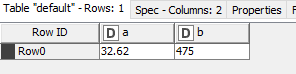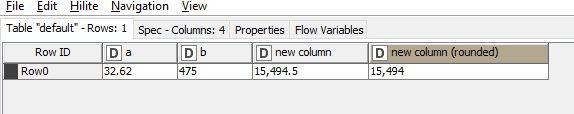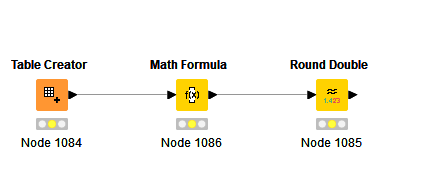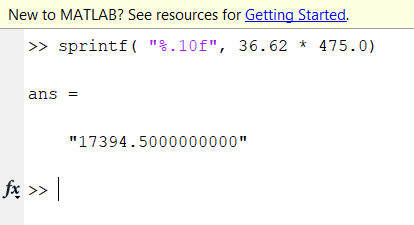# Round half_up

Hi

I try to do simple round of a*b, if decimal is >= 0.5 then round up, if it less than 0.5 round down. I expected the result to be 15495, but somehow the result show 15494.

Do you have any suggestion of what I did wrong?[question.knwf|attachment]1 Like

Hi @kengdeb

Thanks & regards

Ael

question.knwf (8.0 KB)

Sorry for that, hope this time work.

1 Like

The mock up workflow is as simple as this.1 Like

Hi @kengdeb

I was myself surprised and biased thinking that the result was wrong.

The problem is the following:

32.62 times 475 is not 15494.5 but 15494.49999999…

If you change the visualisation to Full Precision as showed in the snapshot above, you will see that this is the case. The Standard Double visualization by default is sometimes misleading in the case of “periodic decimals” or irrational numbers.

The HALF_UP function hence provides the expected result:

HALP_UP( 15494.49999999…) = 15494

HALP_UP( 15494.5) = 15495

Hope this helps.

Best

Ael

4 Likes

Hi @kengdeb

Complementary to my previous message, this problem with double numbers is not a problem with KNIME, but it is due to the way double numbers are coded and handled in binary format by computers. Often computers introduce tiny (0.0000000…0001) errors when converting and operating decimal numbers into binary format which may eventually lead to rounding errors. So for instance here:

32.62 times 475 is definitely 15494.5 for a human

but

32.62 times 475 is 15494.49999 (periodic) for a computer. It doesn’t help when using mathematical truncation or rounding functions, specially when you are at the edge of the truncation or rounding value.

In your case, a possible solution to implement, if you know the maximum number of decimals in your double number, is the following:

If for instance you are handling currency double numbers with cents (two decimals), then you could multiple each of your numbers by 100 and eventually divide the total by 10000. The result is then correct because binary conversion will not need to handle decimals:

The machine result should be

HALF_UP( ((32.62 * 100) * (475 *100)) / 10000) = 5494.5

Hope this helps.

Best

Ael

4 Likes

@aworker

It is quite difficult to explain the reason to business user since it is quite counter intuitive comparing to something like Excel. However, thanks a lot for your details explanation and work around, it works perfectly with my case.

2 Likes

Hi @kengdeb

Thanks for your feedback and for validating the solution.

I fully agree with you. For instance, in Excel:

In Matlab:In Python:

So I guess these other programming languages handle this and amend the rounding issue better at an upper level …

Best

Ael

2 Likes

I tested too in Java and it is the same, the result is correct.

On top of what I just said, I did further research and found the following thread:

RInt differs from the Round class in that it uses the Math.rint() method rather than Math.round(). Unlike Math.round(), in the case of a tie (e.g 1.5) Math.rint() prefers the nearest even integer (so rint(1.5) = 2). This has better statistical properties. For the two argument version you can consider it as a rounding method with a mode of round-half-even.

Apparently KNIME has adopted a -RInt- rounding scheme (or banking Scheme) to avoid “benefit or loss bias” in the long term when rounding. This is not intuitive and obvious to be understood.

Hope this clarifies possible issues or differences too.

Best

Ael

3 Likes

This topic was automatically closed 7 days after the last reply. New replies are no longer allowed.